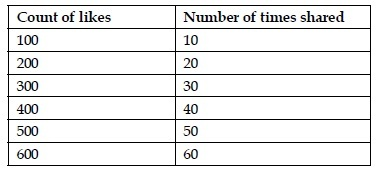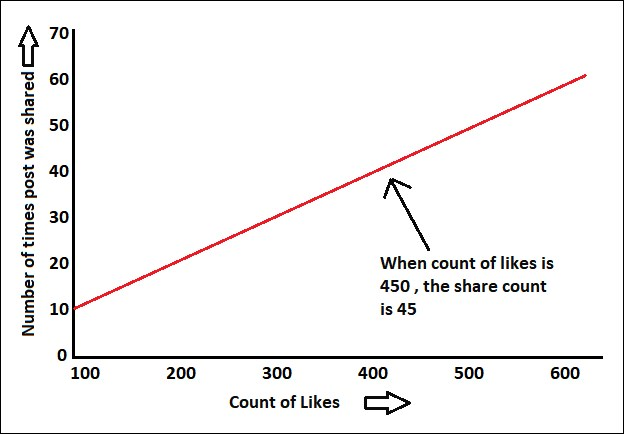•#### Big Data Analytics with Java#### Overview of this book

This book covers case studies such as sentiment analysis on a tweet dataset, recommendations on a movielens dataset, customer segmentation on an ecommerce dataset, and graph analysis on actual flights dataset. This book is an end-to-end guide to implement analytics on big data with Java. Java is the de facto language for major big data environments, including Hadoop. This book will teach you how to perform analytics on big data with production-friendly Java. This book basically divided into two sections. The first part is an introduction that will help the readers get acquainted with big data environments, whereas the second part will contain a hardcore discussion on all the concepts in analytics on big data. It will take you from data analysis and data visualization to the core concepts and advantages of machine learning, real-life usage of regression and classification using Naïve Bayes, a deep discussion on the concepts of clustering,and a review of simple neural networks on big data using deepLearning4j or plain Java Spark code. This book is a must-have book for Java developers who want to start learning big data analytics and want to use it in the real world.
Big Data Analytics with JavaCreditswww.PacktPub.comCustomer FeedbackPrefaceFree Chapter
Big Data Analytics with JavaFirst Steps in Data AnalysisData VisualizationBasics of Machine LearningRegression on Big DataNaive Bayes and Sentiment AnalysisDecision TreesEnsembling on Big DataRecommendation SystemsClustering and Customer Segmentation on Big DataMassive Graphs on Big DataReal-Time Analytics on Big DataDeep Learning Using Big DataIndex## Linear regression

As we mentioned earlier, regression is a technique for predicting continuous values based on certain inputs or variables. With linear regression, we try to learn from data that can fit into a straight linear line. For example, we can try to predict the amount in sales of a product based on variables such as amount spent on advertising, number of hits received on the e-commerce website, price of the product, and percentage offered in terms of sale price. To explain linear regression let's use a simple example using a sample fictitious data of the count of likes on a Facebook post versus the number of times it was shared, as shown in the following table:Let's try to plot this data on a line chart:As you can see in the preceding figure, the data points are linear, which means that they linearly go up. In other words, an independent variable count of likes, when changed causes the value of a dependent variable that is number of shares to change. Thus, if we know a future value...